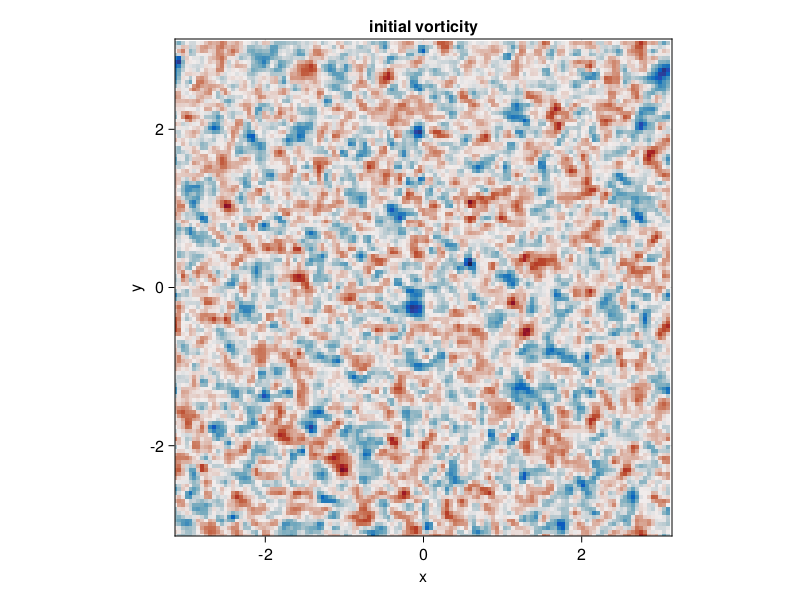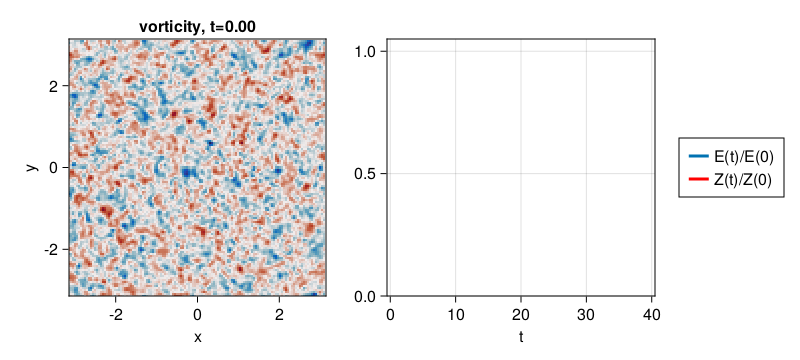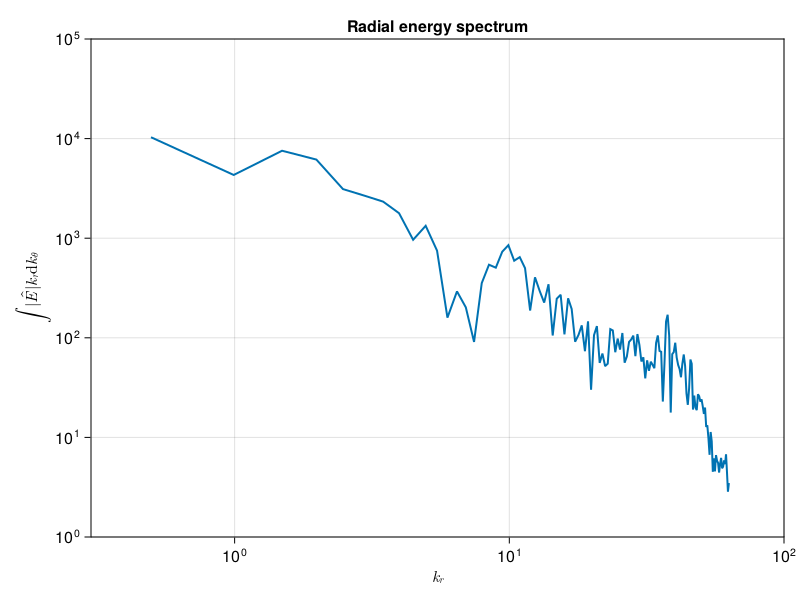# 2D decaying turbulence

This example can be viewed as a Jupyter notebook via.

A simulation of decaying two-dimensional turbulence.

## Install dependencies

First let's make sure we have all required packages installed.

using Pkg
pkg"add GeophysicalFlows, CairoMakie"

## Let's begin

Let's load GeophysicalFlows.jl and some other packages we need.

using GeophysicalFlows, Printf, Random, CairoMakie

using Random: seed!
using GeophysicalFlows: peakedisotropicspectrum

## Choosing a device: CPU or GPU

dev = CPU()     # Device (CPU/GPU)

## Numerical, domain, and simulation parameters

First, we pick some numerical and physical parameters for our model.

n, L  = 128, 2π             # grid resolution and domain length

Then we pick the time-stepper parameters

    dt = 1e-2  # timestep
nsteps = 4000  # total number of steps
nsubs = 20    # number of steps between each plot

## Problem setup

We initialize a Problem by providing a set of keyword arguments. We use stepper = "FilteredRK4". Filtered timesteppers apply a wavenumber-filter at every time-step that removes enstrophy at high wavenumbers and, thereby, stabilize the problem, despite that we use the default viscosity coefficient ν=0.

prob = TwoDNavierStokes.Problem(dev; nx=n, Lx=L, ny=n, Ly=L, dt, stepper="FilteredRK4")

Next we define some shortcuts for convenience.

sol, clock, vars, grid = prob.sol, prob.clock, prob.vars, prob.grid
x,  y  = grid.x,  grid.y
Lx, Ly = grid.Lx, grid.Ly

## Setting initial conditions

Our initial condition tries to reproduce the initial condition used by McWilliams (JFM, 1984).

seed!(1234)
k₀, E₀ = 6, 0.5
ζ₀ = peakedisotropicspectrum(grid, k₀, E₀, mask=prob.timestepper.filter)
TwoDNavierStokes.set_ζ!(prob, ζ₀)

Let's plot the initial vorticity field. Note that when plotting, we decorate the variable to be plotted with Array() to make sure it is brought back on the CPU when vars live on the GPU.

fig = Figure()
ax = Axis(fig[1, 1];
xlabel = "x",
ylabel = "y",
title = "initial vorticity",
aspect = 1,
limits = ((-L/2, L/2), (-L/2, L/2)))

heatmap!(ax, x, y, Array(vars.ζ');
colormap = :balance, colorrange = (-40, 40))

fig## Diagnostics

Create Diagnostics – energy and enstrophy functions are imported at the top.

E = Diagnostic(TwoDNavierStokes.energy, prob; nsteps)
Z = Diagnostic(TwoDNavierStokes.enstrophy, prob; nsteps)
diags = [E, Z] # A list of Diagnostics types passed to "stepforward!" will  be updated every timestep.

## Output

We choose folder for outputing .jld2 files and snapshots (.png files).

filepath = "."
plotpath = "./plots_decayingTwoDNavierStokes"
plotname = "snapshots"
filename = joinpath(filepath, "decayingTwoDNavierStokes.jld2")

Do some basic file management

if isfile(filename); rm(filename); end
if !isdir(plotpath); mkdir(plotpath); end

And then create Output

get_sol(prob) = prob.sol # extracts the Fourier-transformed solution
get_u(prob) = irfft(im * prob.grid.l .* prob.grid.invKrsq .* prob.sol, prob.grid.nx)

out = Output(prob, filename, (:sol, get_sol), (:u, get_u))
saveproblem(out)

## Visualizing the simulation

We initialize a plot with the vorticity field and the time-series of energy and enstrophy diagnostics.

ζ = Observable(Array(vars.ζ))
title_ζ = Observable("vorticity, t=" * @sprintf("%.2f", clock.t))

energy = Observable(Point2f[(E.t, E.data / E.data)])
enstrophy = Observable(Point2f[(Z.t, Z.data / Z.data)])

fig = Figure(resolution = (800, 360))

axζ = Axis(fig[1, 1];
xlabel = "x",
ylabel = "y",
title = title_ζ,
aspect = 1,
limits = ((-L/2, L/2), (-L/2, L/2)))

ax2 = Axis(fig[1, 2],
xlabel = "t",
limits = ((-0.5, 40.5), (0, 1.05)))

heatmap!(axζ, x, y, ζ;
colormap = :balance, colorrange = (-40, 40))

hE = lines!(ax2, energy; linewidth = 3)
hZ = lines!(ax2, enstrophy; linewidth = 3, color = :red)
Legend(fig[1, 3], [hE, hZ], ["E(t)/E(0)", "Z(t)/Z(0)"])

fig## Time-stepping the Problem forward

We time-step the Problem forward in time.

startwalltime = time()

record(fig, "twodturb.mp4", 0:Int(nsteps/nsubs), framerate = 18) do j
if j % (1000 / nsubs) == 0
cfl = clock.dt * maximum([maximum(vars.u) / grid.dx, maximum(vars.v) / grid.dy])

log = @sprintf("step: %04d, t: %d, cfl: %.2f, ΔE: %.4f, ΔZ: %.4f, walltime: %.2f min",
clock.step, clock.t, cfl, E.data[E.i]/E.data, Z.data[Z.i]/Z.data, (time()-startwalltime)/60)

println(log)
end

ζ[] = vars.ζ

energy[] = push!(energy[], Point2f(E.t[E.i], E.data[E.i] / E.data))
enstrophy[] = push!(enstrophy[], Point2f(Z.t[E.i], Z.data[Z.i] / Z.data))

title_ζ[] = "vorticity, t=" * @sprintf("%.2f", clock.t)

stepforward!(prob, diags, nsubs)
TwoDNavierStokes.updatevars!(prob)
end
step: 0000, t: 0, cfl: 0.47, ΔE: 1.0000, ΔZ: 1.0000, walltime: 0.00 min
step: 1000, t: 10, cfl: 0.38, ΔE: 0.9687, ΔZ: 0.1880, walltime: 0.06 min
step: 2000, t: 20, cfl: 0.34, ΔE: 0.9655, ΔZ: 0.1084, walltime: 0.11 min
step: 3000, t: 30, cfl: 0.51, ΔE: 0.9645, ΔZ: 0.0831, walltime: 0.16 min
step: 4000, t: 40, cfl: 0.46, ΔE: 0.9642, ΔZ: 0.0734, walltime: 0.21 min

After the simulation is done we plot the instantaneous radial energy spectrum to illustrate how FourierFlows.radialspectrum can be used,

E  = @. 0.5 * (vars.u^2 + vars.v^2)  # energy density
Eh = rfft(E)                         # Fourier transform of energy density

# compute radial specturm of Eh
kr, Ehr = FourierFlows.radialspectrum(Eh, grid, refinement = 1)

and we plot it.

lines(kr, vec(abs.(Ehr));
linewidth = 2,
axis = (xlabel = L"k_r",
ylabel = L"\int |\hat{E}| k_r \mathrm{d}k_\theta",
xscale = log10,
yscale = log10,
limits = ((0.3, 1e2), (1e0, 1e5))))